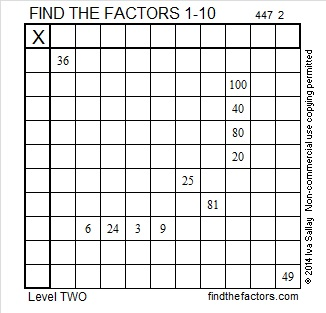# 304 and Level 2

• 304 is a composite number.
• Prime factorization: 304 = 2 x 2 x 2 x 2 x 19, which can be written 304 = (2^4) x 19
• The exponents in the prime factorization are 4 and 1. Adding one to each and multiplying we get (4 + 1)(1 + 1) = 5 x 2 = 10. Therefore 304 has exactly 10 factors.
• Factors of 304: 1, 2, 4, 8, 16, 19, 38, 76, 152, 304
• Factor pairs: 304 = 1 x 304, 2 x 152, 4 x 76, 8 x 38, or 16 x 19
• Taking the factor pair with the largest square number factor, we get √304 = (√16)(√19) = 4√19 ≈ 17.436This 1 – 10 multiplication table has only twelve clues. Can you still complete the entire table?Print the puzzles or type the factors on this excel file: 10 Factors 2014-11-24This site uses Akismet to reduce spam. Learn how your comment data is processed.